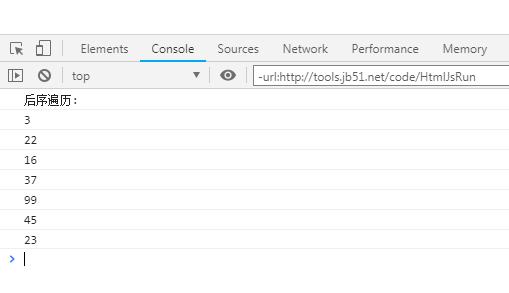JavaScript数据结构与算法之二叉树遍历算法详解【先序、中序、后序】

更新时间：2019年02月21日 14:17:07   作者：白杨-M我要评论

javascript数据结构与算法--二叉树遍历（先序）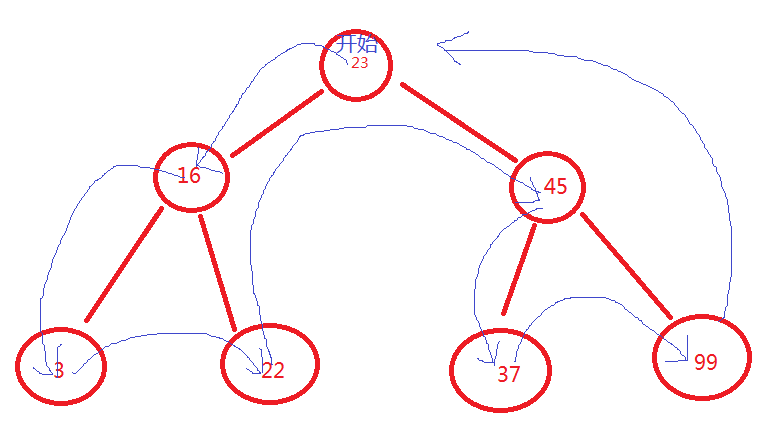/*
*二叉树中，相对较小的值保存在左节点上，较大的值保存在右节点中
*
*
* */
/*用来生成一个节点*/
function Node(data, left, right) {
this.data = data;//节点存储的数据
this.left = left;
this.right = right;
this.show = show;
}
function show() {
return this.data;
}
/*用来生成一个二叉树*/
function BST() {
this.root = null;
this.insert = insert;
}
/*将数据插入二叉树
（1）设根节点为当前节点。
（2）如果待插入节点保存的数据小于当前节点，则设新的当前节点为原节点的左节点；反
之，执行第4步。
（3）如果当前节点的左节点为null，就将新的节点插入这个位置，退出循环；反之，继续
执行下一次循环。
（4）设新的当前节点为原节点的右节点。
（5）如果当前节点的右节点为null，就将新的节点插入这个位置，退出循环；反之，继续
执行下一次循环。
* */
function insert(data) {
var n = new Node(data, null, null);
if (this.root == null) {
this.root = n;
}
else {
var current = this.root;
var parent;
while (true) {
parent = current;
if (data < current.data) {
current = current.left;//待插入节点保存的数据小于当前节点，则设新的当前节点为原节点的左节点
if (current == null) {//如果当前节点的左节点为null，就将新的节点插入这个位置，退出循环；反之，继续执行下一次while循环。
parent.left = n;
break;
}
}
else {
current = current.right;//待插入节点保存的数据小于当前节点，则设新的当前节点为原节点的左节点
if (current == null) {
parent.right = n;
break;
}
}
}
}
}
/*先序遍历
*用递归的方法
*/
function preOrder(node) {
if (!(node == null)) {
console.log(node.show() + " ");
preOrder(node.left);
preOrder(node.right);
}
}
/* 测试代码 */
var nums = new BST();
nums.insert(23);
nums.insert(45);
nums.insert(16);
nums.insert(37);
nums.insert(3);
nums.insert(99);
nums.insert(22);
console.log("先序遍历: ");
preOrder(nums.root);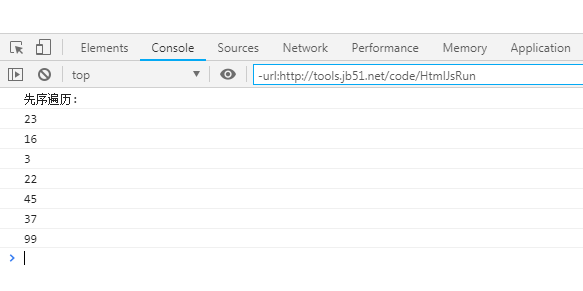javascript数据结构与算法--二叉树遍历（中序）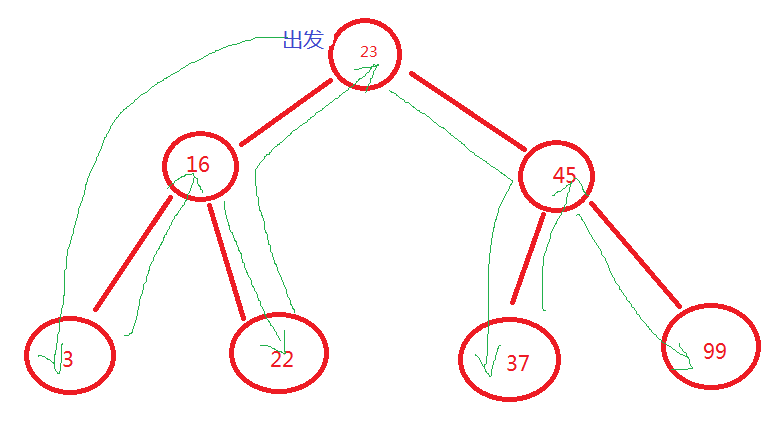/*
*二叉树中，相对较小的值保存在左节点上，较大的值保存在右节点中
*
*
* */
/*用来生成一个节点*/
function Node(data, left, right) {
this.data = data;//节点存储的数据
this.left = left;
this.right = right;
this.show = show;
}
function show() {
return this.data;
}
/*用来生成一个二叉树*/
function BST() {
this.root = null;
this.insert = insert;
}
/*将数据插入二叉树
（1）设根节点为当前节点。
（2）如果待插入节点保存的数据小于当前节点，则设新的当前节点为原节点的左节点；反
之，执行第4步。
（3）如果当前节点的左节点为null，就将新的节点插入这个位置，退出循环；反之，继续
执行下一次循环。
（4）设新的当前节点为原节点的右节点。
（5）如果当前节点的右节点为null，就将新的节点插入这个位置，退出循环；反之，继续
执行下一次循环。
* */
function insert(data) {
var n = new Node(data, null, null);
if (this.root == null) {
this.root = n;
}
else {
var current = this.root;
var parent;
while (true) {
parent = current;
if (data < current.data) {
current = current.left;//待插入节点保存的数据小于当前节点，则设新的当前节点为原节点的左节点
if (current == null) {//如果当前节点的左节点为null，就将新的节点插入这个位置，退出循环；反之，继续执行下一次while循环。
parent.left = n;
break;
}
}
else {
current = current.right;//待插入节点保存的数据小于当前节点，则设新的当前节点为原节点的左节点
if (current == null) {
parent.right = n;
break;
}
}
}
}
}
/*中序遍历
*用递归的方法
*/
function inOrder(node) {
if (!(node == null)) {
inOrder(node.left);
console.log(node.show() + " ");
inOrder(node.right);
}
}
/* 测试代码 */
var nums = new BST();
nums.insert(23);
nums.insert(45);
nums.insert(16);
nums.insert(37);
nums.insert(3);
nums.insert(99);
nums.insert(22);
console.log("中序遍历: ");
inOrder(nums.root);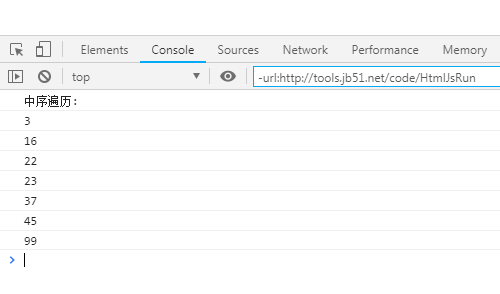javascript数据结构与算法--二叉树遍历（后序）

/*
*二叉树中，相对较小的值保存在左节点上，较大的值保存在右节点中
*
*
* */
/*用来生成一个节点*/
function Node(data, left, right) {
this.data = data;//节点存储的数据
this.left = left;
this.right = right;
this.show = show;
}
function show() {
return this.data;
}
/*用来生成一个二叉树*/
function BST() {
this.root = null;
this.insert = insert;
}
/*将数据插入二叉树
（1）设根节点为当前节点。
（2）如果待插入节点保存的数据小于当前节点，则设新的当前节点为原节点的左节点；反
之，执行第4步。
（3）如果当前节点的左节点为null，就将新的节点插入这个位置，退出循环；反之，继续
执行下一次循环。
（4）设新的当前节点为原节点的右节点。
（5）如果当前节点的右节点为null，就将新的节点插入这个位置，退出循环；反之，继续
执行下一次循环。
* */
function insert(data) {
var n = new Node(data, null, null);
if (this.root == null) {
this.root = n;
}
else {
var current = this.root;
var parent;
while (true) {
parent = current;
if (data < current.data) {
current = current.left;//待插入节点保存的数据小于当前节点，则设新的当前节点为原节点的左节点
if (current == null) {//如果当前节点的左节点为null，就将新的节点插入这个位置，退出循环；反之，继续执行下一次while循环。
parent.left = n;
break;
}
}
else {
current = current.right;//待插入节点保存的数据小于当前节点，则设新的当前节点为原节点的左节点
if (current == null) {
parent.right = n;
break;
}
}
}
}
}
/*后序遍历
*用递归的方法
*/
function postOrder(node) {
if (!(node == null)) {
postOrder(node.left);
postOrder(node.right);
console.log(node.show() + " ");
}
}
/* 测试代码 */
var nums = new BST();
nums.insert(23);
nums.insert(45);
nums.insert(16);
nums.insert(37);
nums.insert(3);
nums.insert(99);
nums.insert(22);
console.log("后序遍历: ");
postOrder(nums.root);